# Parametric continuous distributions

Tip

To produce the example graphs of distributions below, we used a sample size of 1000, equal sample probability steps, samples per PDF of 10, and we set the graph style to line. Even if you use the same options, your graphs can look slightly different due to random variation in the Monte Carlo sampling.

## Uniform(min, max)

Uniform distribution (known also as a rectangular distribution), is a distribution that has constant probability (all intervals of the same length on the distribution's support are equally probable).

Uniform distribution can be created in Analytica by the Uniform function for values between «min» and «max». If omitted, they default to 0 and 1. If you specify optional parameter Integer: True, it returns a discrete distribution consisting of only the integers between «min» and «max», each with equal probability. See Uniform(min, max, Integer: True) and Uniform().

When to use: If you know nothing about the uncertain quantity other than its bounds, a uniform distribution between the bounds is appealing. However, situations in which this is truly appropriate are rare. Usually, you know that one end or the middle of the range is more likely than the rest — that is, the quantity has a mode. In such cases, a beta or triangular distribution is a better choice.

Example:

Uniform(5, 10) →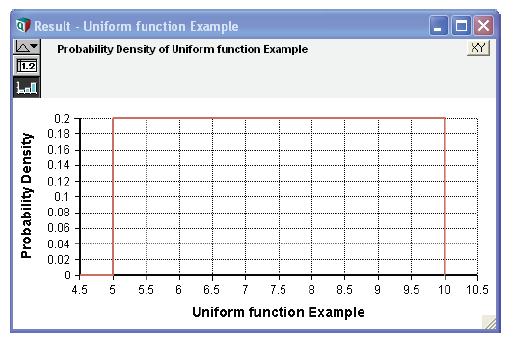## Triangular(min, mode, max)

Creates a triangular distribution, with minimum «min», most likely value «mode», and maximum «max». «min» must not be greater than «mode», and mode must not be greater than «max». See Triangular.

When to use: Use the triangular distribution when you have the bounds and the mode, but have little other information about the uncertain quantity.

Example:

Triangular(2, 7, 10) →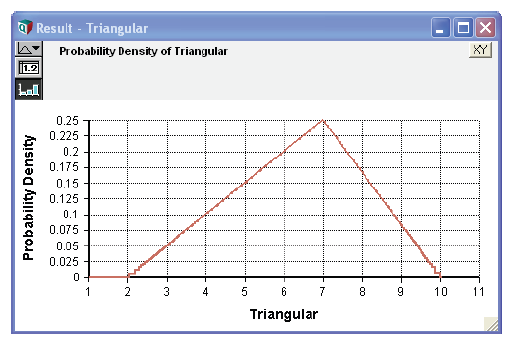## UncertainLMH( xLow, xMedian, xHigh, pLow, lb, ub )

(Requires Analytica 5.0 or later)

A smooth continuous distribution from 10-50-90 percentiles (or other symmetric percentiles), which can be either an unbounded, semi-bounded or full-bounded distribution depending on whether «lb» or «ub» is specified. Use the optional 0 < «pLow» < 50% parameter when specifying other symmetric percentile levels.

When to use: Use when you have only low-median-high estimates for a continuous quantity, with no external rationale for a specific distributional shape. Preferred to Triangular when tails are possible in either direction.

Examples:

UncertainLMH(2, 7, 10)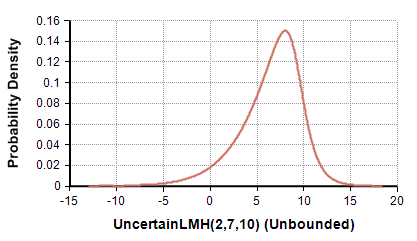UncertainLMH(2,7,10, lb:0)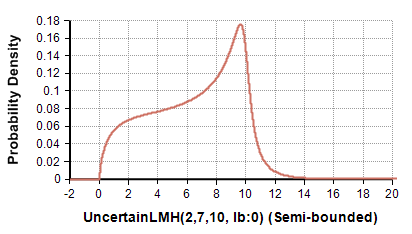## Normal(mean, stddev)

Creates a normal or Gaussian probability distribution with «mean» and standard deviation «stddev». The standard deviation must be 0 or greater. The range [«mean» - «stddev», «mean» + «stddev»] encloses about 68% of the probability. See also Normal().

When to use: Use a normal distribution if the uncertain quantity is unimodal and symmetric and the upper and lower bounds are unknown, possibly very large or very small (unbounded). This distribution is particularly appropriate if you believe that the uncertain quantity is the sum or average of a large number of independent, random quantities.

Example:

Normal(30, 5) →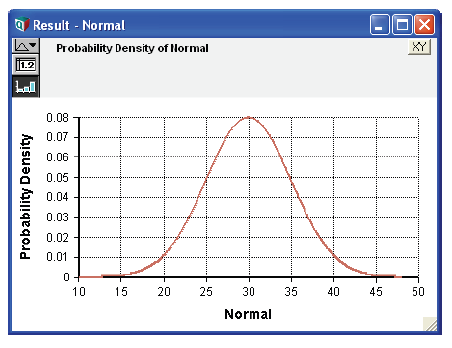## LogNormal(median, gsdev, mean, stddev)

Creates a lognormal distribution. You can specify its median and geometric standard deviation «gsdev», or its mean and standard deviation «stddev», or any two of these four parameters. The geometric standard deviation, «gsdev», must be 1 or greater. It is sometimes also known as the uncertainty factor or error factor. The range [«median»/«gsdev», «median» x «gsdev»] encloses about 68% of the probability — just like the range [«mean» - «stddev», «mean» + «stddev»] for a normal distribution with standard deviation «stddev». «median» and «gsdev» must be positive. See also Lognormal().

If «x» is lognormal Ln(x) has a normal distribution with mean Ln(median) and standard deviation Ln(gsdev).

When to use: Use the lognormal distribution if you have a sharp lower bound of zero but no sharp upper bound, a single mode, and a positive skew. The gamma distribution is also an option in this case. The lognormal is particularly appropriate if you believe that the uncertain quantity is the product (or ratio) of a large number of independent random variables. The multiplicative version of the central limit theorem says that the product or ratio of many independent variables tends to lognormal — just as their sum tends to a normal distribution.

Examples:

Lognormal(5, 2) →
Lognormal(mean: 6.358, Stddev: 5) →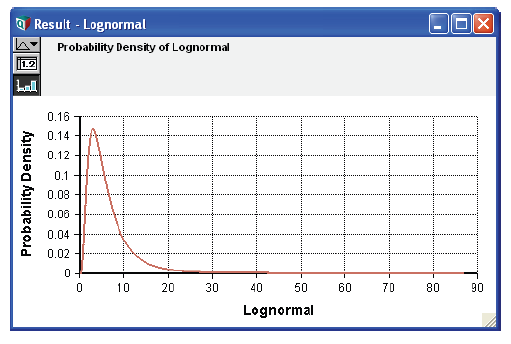## Beta(x, y, min, max)

Creates a beta distribution of numbers between 0 and 1 if you omit optional parameters «min» and «max». «x» and «y» must be positive. If you specify «min» and/or «max», it shifts and expands the beta distribution to so that they form the lower and upper bounds. See also Beta(). The mean is:

$\displaystyle{ \frac{x}{x + y} \times (max - min) + min }$

When to use: Use a beta distribution to represent uncertainty about a continuous quantity bounded by 0 and 1 (0% or 100%) with a single mode. It is particularly useful for modeling an opinion about the fraction (percentage) of a population that has some characteristic. For example, suppose you are trying to estimate the long run frequency of heads, h, for a bent coin about which you know nothing. You could represent your prior opinion about h as a uniform distribution:

Uniform(0, 1)

Or equivalently:

Beta(1, 1)

If you observe r heads in n tosses of the coin, your new (posterior) opinion about h, should be:

Beta(1 + r, 1 + n - r)

If the uncertain quantity has lower and upper bounds other than 0 and 1, include the lower and upper bounds parameters to obtain a transformed beta distribution. The transformed beta is a very flexible distribution for representing a wide variety of bounded quantities.

Examples:

Beta(5, 10) →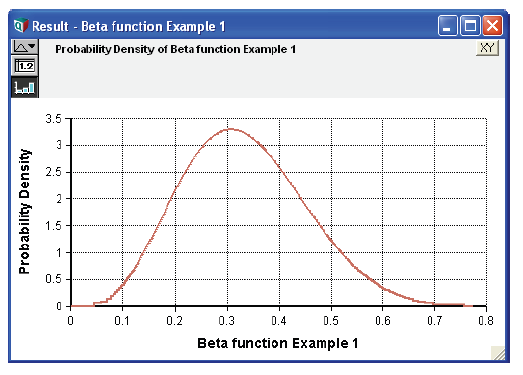Beta(5, 10, 2, 4) →## Exponential(r)

Describes the distribution of times between successive independent events in a Poisson process with an average rate of «r» events per unit time. The rate «r» is the reciprocal of the mean of the Poisson distribution — the average number of events per unit time. Its standard deviation is also 1/«r». See also Exponential().

A model with exponentially distributed times between events is said to be Markov, implying that knowledge about when the next event occurs does not depend on the system’s history or how much time has elapsed since the previous event. More general distributions such as the gamma or Weibull do not exhibit this property.

Example:

Exponential(10) →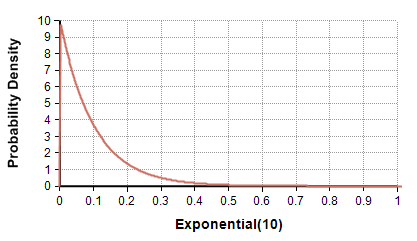## Gamma(a, b)

Creates a gamma distribution with shape parameter «a» and scale parameter «b». The scale parameter, «b», is optional and defaults to «b»=1. The gamma distribution is bounded below by zero (all sample points are positive) and is unbounded from above. It has a theoretical mean of $\displaystyle{ a \cdot b }$ and a theoretical variance of $\displaystyle{ a \cdot b^2 }$ . When «a» > «b» , the distribution is unimodal with the mode at $\displaystyle{ (a - 1) \cdot b }$ . An exponential distribution results when «a» = 1 . As $\displaystyle{ a \to \infty }$ , the gamma distribution approaches a normal distribution in shape.

The gamma distribution encodes the time required for «a» events to occur in a Poisson process with mean arrival time of «b». See also Gamma().

Tip

Some textbooks use Rate=1/«b», instead of «b», as the scale parameter.

When to use: Use the gamma distribution with «a» >1 if you have a sharp lower bound of zero but no sharp upper bound, a single mode, and a positive skew. The Lognormal distribution is also an option in this case. Gamma is especially appropriate when encoding arrival times for sets of events. A gamma distribution with a large value for a is also useful when you wish to use a bell-shaped curve for a positive-only quantity.

Examples: Gamma distributions with mean = 1## Logistic(m, s)

The logistic distribution describes a distribution with a cumulative density given by:

$\displaystyle{ F(x) = \frac{1}{1 + e^{ \frac{-(x - m)}{s}}} }$

The distribution is symmetric and unimodal with tails that are heavier than the normal distribution. It has a mean and mode of «m», variance of:

$\displaystyle{ \frac{\pi^2 \times s^2}{3} }$

and kurtosis of 6/5 and no skew. The scale parameter, «s», is optional and defaults to 1.

The logistic distribution is particularly convenient for determining dependent probabilities using linear regression techniques, where the probability of a binomial event depends monotonically on a continuous variable x. For example, in a toxicology assay, x might be the dosage of a toxin, and p(x) the probability of death for an animal exposed to that dosage. Using p(x) = F(x), the logit of p, given by:

Logit(p(x)) = Ln(p(x) / (1-p(x))) = x/s - m/s

This has a simple linear form. This linear form lends itself to linear regression techniques for estimating the distribution — for example, from clinical trial data. See also Logistic().

Example:

Logistic(10, 10)## StudentT(d)

The StudentT() describes the distribution of the deviation of a sample mean from the true mean when the samples are generated by a normally distributed process centered on the true mean. The T statistic is:

T = (m - x)/(s Sqrt(n))

where «x» is the sample mean, «m» is the actual mean, «s» is the sample standard deviation, and «n» is the sample size. T is distributed according to StudentT with «d» = n-1 degrees of freedom.

The StudentT distribution is often used to test the statistical hypothesis that a sample mean is significantly different from zero. If x1 .. xn measurements are taken to test the hypothesis m > 0:

GetFract(StudentT(n - 1), 0.95)

This is the acceptance threshold for the T statistic. If T is greater than this fractile, we can reject the null hypothesis (that «m» <= 0) at 95% confidence. When using GetFract for hypothesis testing, be sure to use a large sample size, since the precision of this computation improves with sample size.

The StudentT can also be useful for modeling the power of hypothetical experiments as a function of the sample size n, without having to model the outcomes of individual trials.

Example:

StudentT(8)## Weibull(n, s)

The Weibull distribution has a cumulative density given by:

$\displaystyle{ f(x) = 1 - e^{-(\frac{x}{s})^n} }$

for «x» >= 0. It is similar in shape to the gamma distribution, but tends to be less skewed and tailheavy. It is often used to represent failure time in reliability models. In such models, might represent the proportion of devices that experience a failure within the first «x» time units of operation, the number of insurance policy holders that file a claim within «x» days. See also Weibull.

Example:

Weibull(10, 4) →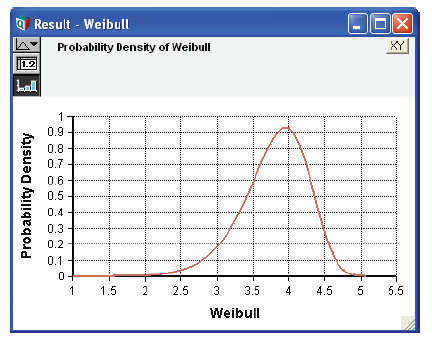## ChiSquared(d)

The ChiSquared distribution with «d» degrees of freedom describes the distribution of a Chi-Squared metric defined as:

$\displaystyle{ Chi^2 = \sum_{i=1}^n y_{i}^2 }$

where each yi is independently sampled from a standard normal distribution and «d» = «n» -1. The distribution is defined over non-negative values.

The Chi-squared distribution is commonly used for analyses of second moments, such as analyses of variance and contingency table analyses. It can also be used to generate the F distribution. Suppose:

Variable V := ChiSquared(k)
Variable W := ChiSquared(m)
Variable S := (V/k)*(W/m)

S is distributed as an F distribution with «k» and «m» degrees of freedom. The F distribution is useful for the analysis of ratios of variance, such as a one-factor between-subjects analysis of variance.